Courses

# Work, Power, Potential Energy and Kinetic Energy Relations (Part - 4) Civil Engineering (CE) Notes | EduRev

## Civil Engineering (CE) : Work, Power, Potential Energy and Kinetic Energy Relations (Part - 4) Civil Engineering (CE) Notes | EduRev

The document Work, Power, Potential Energy and Kinetic Energy Relations (Part - 4) Civil Engineering (CE) Notes | EduRev is a part of Civil Engineering (CE) category.
All you need of Civil Engineering (CE) at this link: Civil Engineering (CE)

4.1.11 Force, Torque and Power curves for actuators and motors

Concepts of power and work are particularly useful to calculate the behavior of a system that is driven by a motor. Calculations like this are described in more detail in Section 4.12. In this section, we discuss the relationships between force, torque, speed and power for motors.

There are many different types of motor, and each type has its own unique characteristics. They all have the following features in common, however:

1. The motors convert some non-mechanical form of energy into mechanical work. For example, an electric motor converts electrical energy; an internal combustion engine, your muscles, and other molecular motors convert chemical energy, and so on.

2. A linear motor or linear actuator applies a force to an object (or more accurately, it applies roughly equal and opposite forces on two objects connected to its ends). Your muscles are good examples of linear actuators. You can buy electrical or hydraulically powered actuators as well.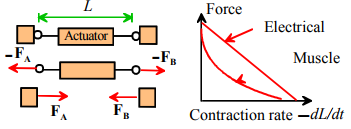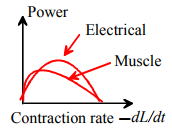3. The forces applied by an actuator depend on (i) the amount of power supplied to the actuator – electrical, chemical, etc; and (ii) the rate of contraction, or stretching, of the actuator. The typical variation of force with stretch rate is shown in the figure. The figure assumes that the actuator pulls on the objects attached to its ends (like a muscle); but some actuators will push rather than pull; and some can do both. If the actuator extends slowly and is not rotating it behaves like a two-force member, and the forces exerted by its two ends are equal and opposite.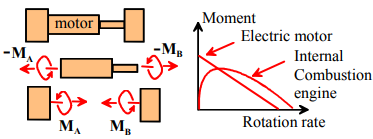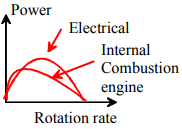4. A rotary motor or rotary actuator applies a moment or torque to an object (or, again, more precisely it applies equal and opposite moments to two objects). Electric motors, internal combustion engines, bacterial motors are good examples of rotary motors.

5. The torques exerted by a rotary actuator depend on (i) the amount of power supplied to the actuator – electrical, chemical, etc; and (ii) the relative velocity of the two objects on which the forces act. The typical variation of force with external power applied and with relative velocity is shown in the figure. An electric motor applies a large torque when it is stationary, and its torque decreases with rotational speed. An internal combustion engine applies no torque when it is stationary. It’s torque is greatest at some intermediate speed, and decreases at high speed.

The power curve describes the variation of the rate of work done by an actuator or motor with its extension or contraction rate or rotational speed. Note that

1. The rate of work done (or power expended) by a linear actuator that applies forces FA.FB to the objects attached to its two ends can be calculated as P = FA . vA + FB . vB, where vA and vB are the velocities of the two ends.

2. The rate of work (or power expended) by a rotational motor that applies moments MA, MB to the objects attached to its two ends is P = MA . ωA + MB . ωB.

Typical power curves for motors and actuators are sketched in the figures.

The efficiency of a motor or actuator is the ratio of the rate of work done by the forces or moments exerted by the motor to the electrical or chemical power. The efficiency is always less than 1 because some fraction of the power supplied to the motor is dissipated as heat. An electric motor has a high efficiency; heat engines such as an internal combustion engine have a much lower efficiency, because they operate by raising the temperature of the air inside the cylinders to increase its pressure. The heat required to increase the temperature can never be completely converted into useful work (EN72 will discuss the reasons for this in more detail). The efficiency of a motor always varies with its speed – there is a special operating speed that maximizes its efficiency. For an internal combustion engine, the speed corresponding to maximum efficiency is usually quite low – 1500rpm or so. So, to minimize fuel consumption during your driving, you need to operate the engine at this speed for as much of your drive as possible.

There is not enough time in this course to be able to discuss the characteristics of actuators and motors in great detail. Instead, we focus on one specific example, which helps to illustrate the general characteristics in more detail.

Torque Curves for a brushed electric motor. There are several different types of electric motor – here, we will focus on the simplest and cheapest – the so called `Brushed DC electric motor.’ The term `Brushed’ refers to the fact that the motor is driven by a coil of wire wrapped around its rotating shaft, and `brushes’ are used to make an electrical contact between the power supply and the rotating shaft. `DC’ means that the motor is driven by direct, rather than alternating current.

Here is a very brief summary of the basic principles of this type of motor. The underlying theory will be discussed in more detail in EN510

• An electric motor applies a moment, or torque to an object that is coupled to its output shaft. The moment is developed by electromagnetic forces acting between permanent magnets in the housing and the electric current flowing through the winding of the motor. In the following discussion, we shall assume that the body of the motor is stationary ωA = 0, and its output shaft rotates with angular velocity ωB = ωn where n is a unit vector parallel to the shaft and ω is its angular speed. In addition, we assume that the output shaft exerts a moment MB = Tn.
•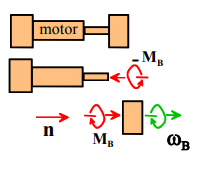• Power to drive the motor is supplied by connecting an electrical power source (e.g. a battery) to its terminals. The power supply generally applies a fixed voltage to the terminals, which then causes current to flow through the winding. The current is proportional to the voltage, and decreases in proportion to the angular (rotational) speed of the output shaft of the motor.
• The moment applied by the output shaft is proportional to this electric current.

The electric current I flowing through the winding is related to the voltage V and angular speed of the motor ω (in radians per second) by

I = (V - βω) / R

where R is the electrical resistance of the winding, and β is a constant that depends on the arrangement and type of magnets used in the motor, as well as the geometry of the wire coil.

The magnitude of moment exerted by output shaft of the motor T is related to the electric current and the speed of the motor by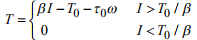where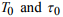are constants that account for losses such as friction in the bearings, eddy currents, and air resistance.

The torque-current and the current-voltage-speed relations can be combined into a single formula relating torque to voltage and motor speed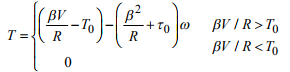This relationship is sketched in the figure – this is called the torque curve for the motor. The two most important points on the curve are

1. The `stall torque’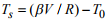2. The `No load’ speed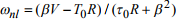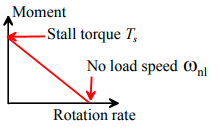The torque curve can be expressed in terms of these quantities as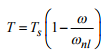Motor manufacturers generally provide values of the stall torque and no load speed for a motor. Some manufacturers provide enough data so that you can usually calculate the values of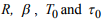for their product.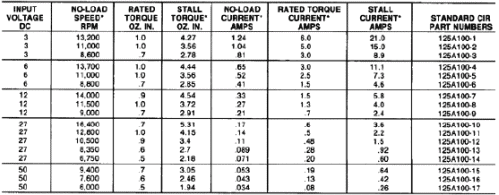Here

1. The `input voltage’ is the recommended voltage V to apply to the motor. All other data in the table assume that the motor is used with this voltage. If you wish, you can run a motor with a lower voltage (this will make it run more slowly) and if you are feeling brave you can increase the voltage slightly – but this might cause it to burn out, invalidate your warranty and expose you to a potential lawsuit from your customers…

2. The `No load speed’ ωnl is the speed of the motor when it is spinning freely with T=0
3. The `No load current’ Inl is the corresponding current
4. The `Stall torque’ Ts is the value of T when the motor is not spinning ω = 0
5. The ‘Stall current’ Is is the corresponding current
6. The `rated torque’ and `rated current’ are limits to the steady running of the motor – the torque and current should not exceed these values for an extended period of time.

The values in the table can be substituted into the formulas relating current, voltage, torque and motor speed, which yield four equations for the unknown values of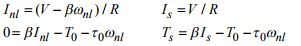Solving these equations gives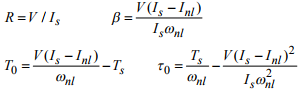HEALTH WARNING: When you use these formulas it is critical to use a consistent set of units. SI units are very strongly recommended! Volts and Amperes are SI units of electrical potential and electric current. But the torques must be converted into Nm. The conversion factor is 1 ‘oz-in’ is 0.0070612 Nm

Not all motor manufacturers provide such detailed specifications – it is more common to be given (i) the stall torque; (ii) the no load speed; and (iii) the stall current. If this is the case you have to assume Inl = 0 and take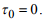If you aren’t given the stall current you have to take T0 = 0 as well.

Power Curves for a brushed electric motor driven from a constant voltage power supply: The rate of work done by the motor is P = MBB = Tω . The power can be calculated in terms of the angular speed of the motor as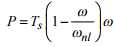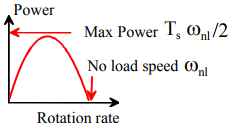The power curve is sketched in the figure. The most important features are (i) The motor develops no power at both zero speed and the no load speed; and (ii) the motor develops its maximum power Pmax = Tsωnl /2 at speed ωnl /2.

Efficiency of a brushed electric motor: The electrical power supplied to a motor can be calculated from the current I and voltage V as Pin = VI. The efficiency can therefore be calculated as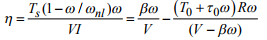The second expression can be used to calculate the speed that maximizes efficiency.

Offer running on EduRev: Apply code STAYHOME200 to get INR 200 off on our premium plan EduRev Infinity!

,

,

,

,

,

,

,

,

,

,

,

,

,

,

,

,

,

,

,

,

,

,

,

,

,

,

,

;